# Learn Embedded Programming [Step by Step]

## Embedded Programming Tutorials for Beginners with Step By Step Guide.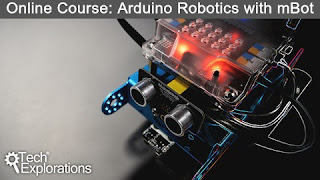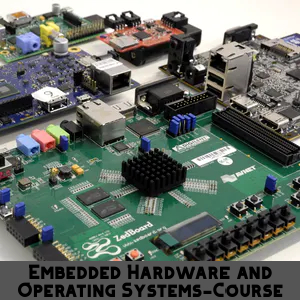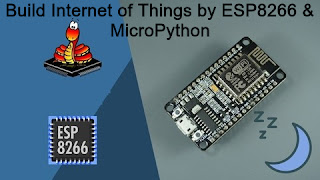## Temperature Meter using Microcontroller and LM35 Temperature Sensor

We can  build a Temperature meter by pic18f2550 microcontroller and we need a temperature sensor . In this project we will use LM35 temperature sensor and it is a popular temperature sensor .Look at the picture which is given below .The Picture has been taken from internet
LM35 produce different voltage outputs at different temperatures . LM35 increase it's output voltage 10mV or 0.01v  for each degree increment of temperature . That means 0.01 v per degree centigrade.
Now we need to get a microcontroller as a system to read temperature from sensor . We will use ADC of the microcontroller and the reading will be shown in LCD display . It's the basic idea of the project .

#### We need a basic knowledge about ADC . Let's  take a look at here :

Basically ADC is like as voltage divider . According to voltage It produce output .
bit 5 : VCFG0: Voltage Reference Configuration bit (VREF- source)
1 = VREF- (AN2)
0 = VSS or 0 volt

bit 4 :VCFG0: Voltage Reference Configuration bit (VREF+ source)
1 = VREF+ (AN3)
0 = VDD or 5volt

We will set  VCFG0[bit 5]=0and VCFG0[bit4]=0 . So we will get highest value 5volt and lowest value 0volt.The ADCON1 is a 10 bit register that means  (2 to the power 10) is it's highest counting capacity and the result is 1024 . So this register can count from 0 to 1023 . When 0 volt , we get reading at RA0 pin  0 .When 5 volt , we get reading at RA0 pin 1023. It means 5volt equivalent to 1023 .
If  1023 reading value    equal   to 5 volt.
So 1      reading value    equal   to  5/1023 volt
As we know LM35 reading can be changed  with  0.01 v per degree centigrade change . According to the datasheet 10mV = 1 degree centigrade temperature .The temperature calculation should be like that :
0.01 volt   for     1      degree centigrade Temperature
so  1     ,,       ,,  (1/0.01)   ,,         ,,              ,,

### Source Code :

``````

sbit LCD_RS at LATB7_bit;
sbit LCD_EN at LATB6_bit;
sbit LCD_D4 at LATB5_bit;
sbit LCD_D5 at LATB4_bit;
sbit LCD_D6 at LATB3_bit;
sbit LCD_D7 at LATB2_bit;

sbit LCD_RS_Direction at TRISB7_bit;
sbit LCD_EN_Direction at TRISB6_bit;
sbit LCD_D4_Direction at TRISB5_bit;
sbit LCD_D5_Direction at TRISB4_bit;
sbit LCD_D6_Direction at TRISB3_bit;
sbit LCD_D7_Direction at TRISB2_bit;
// End LCD module connections

double source=0;
int temperature=0;
char txt;

void main() {
ADCON1=0x0E;                  // Configure RA0 pin as input
CMCON=7;
TRISB.F0=0;

Lcd_Init();                        // Initialize LCD
Lcd_Cmd(_LCD_CLEAR);               // Clear display
Lcd_Cmd(_LCD_CURSOR_OFF);          // Cursor off

Lcd_Out(1, 3, "Temperature");
// Different LCD displays have different
Lcd_Chr(2,12,223);                   //   char code for degree
Lcd_Chr(2,13,'C');                  // Display "C" for Celsius
while(1){
source=(source*5)/1023;
source=source/0.01;
if(source>30){       // when temperature goes higher than 30 degree , fan turns on .
PORTB.F0=1;
}
else{                    // when temperature goes lower than 30 degree , fan remains off .
PORTB.F0=0;
}
inttostr(source,txt);
Lcd_Out(2,1,txt);

}
}

``````

## Water Level Indicator Project using Microcontroller

Water tank controller  is an embedded project where motor switch automatically controlled by a microcontroller .When tank's water level is very low , the microcontroller system turns on the motor switch , Until the water level is full or 100% and the motor switch remains on . When microcontroller get notification tank is full , it turns off the switch of motor . Until the tank is empty this stage remains on .
Now the question is , how can we get notification about water level ? We will apply a technique . Look at the picture given below .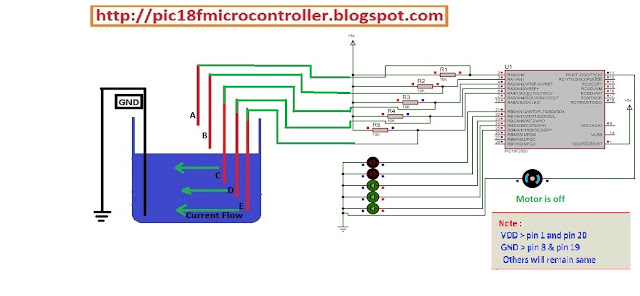Microcontroller Project : Water Tank Controller using pic18f2550 Microcontroller

Here microcontroller pins (RA0-RA4)  are connected to VDD through five 10k Ohm resistors . Five cables are connected to the points between resistors first end points and mcu (RA0-RA4)pin points . Those five (A,B,C,D,E) cables are hanged  into the tank at different five levels(A,B,C,D,E) so that each cable represents each of five levels individually .Microcontroller Project : Water Tank Controller using pic18f2550 Microcontroller
Once water level goes to E level , it makes enable the connection between GND and 'E' level cable . So , it makes the RA4 pin GND(logical 0) and we get notification the tank is empty . Consequently the system turns on the motor of switch .Water Tank Controller Project using Microcontroller

When it's full, all the mcu pins(RA0-RA4) goes to low or 0 . We get the notification tank is full the motor needs to be turned off . That's the working process of this system.

### Souece Code :

``````

void main() {
ADCON1=0x0F;                 // Configure RA) pin as input
CMCON=7;
TRISC.F0=0;
TRISA.F0=1;
TRISA.F1=1;
TRISA.F2=1;
TRISA.F3=1;
TRISA.F4=1;
TRISB=0x00;
while(1){
if(PORTA.F4==0 && PORTA.F3==0 && PORTA.F2==0 &&  PORTA.F1==0 && PORTA.F0==0){
PORTB.F0=1;
PORTB.F1=1;                                         // 100% full
PORTB.F2=1;
PORTB.F3=1;
PORTB.F4=1;
PORTC.F0=0;   //motor turned off
}
if(PORTA.F4==0 && PORTA.F3==0 && PORTA.F2==0 &&  PORTA.F1==0 && PORTA.F0==1){
PORTB.F0=0;
PORTB.F1=1;                                        // 80% full
PORTB.F2=1;
PORTB.F3=1;
PORTB.F4=1;}
if(PORTA.F4==0 && PORTA.F3==0 && PORTA.F2==0 &&  PORTA.F1==1 && PORTA.F0==1){
PORTB.F0=0;
PORTB.F1=0;
PORTB.F2=1;                                            // 60% full
PORTB.F3=1;
PORTB.F4=1;

}
if(PORTA.F4==0 && PORTA.F3==0 && PORTA.F2==1 &&  PORTA.F1==1 && PORTA.F0==1){
PORTB.F0=0;
PORTB.F1=0;                                            // 40% full
PORTB.F2=0;
PORTB.F3=1;
PORTB.F4=1;
}
if(PORTA.F4==0 && PORTA.F3==1 && PORTA.F2==1 &&  PORTA.F1==1 && PORTA.F0==1){
PORTB.F0=0;
PORTB.F1=0;
PORTB.F2=0;
PORTB.F3=0;                                              // 20% full
PORTB.F4=1;
PORTC.F0=1;   //motor turned on
}
}
}``````

### Circuit :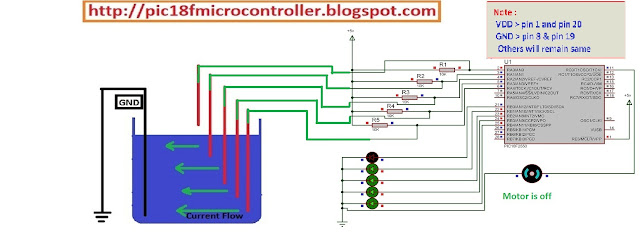Water Tank Controller Project using MicrocontrollerWater Tank Controller Project using Microcontroller

## Battery Charge Level Indicator (5volt) using Microcontroller

Battery Charge Level Viewer is a microcontroller based small project which shows charge level of battery using 5 LEDs . Generally battery becomes full at 5volts . Remember that an ADC channel of pic18f2550 can count from 0 to 1023 . Because it's ADC channel register is 10bits. As we like to  measure 5volt  . We will read the value of adc channel and will convert it to voltage . After converting into voltage we get a understandable value and we shows it through 5 green LEDs. It's the basic concept .

### ADC(Analog to Digital Converter ) :

We need a basic knowledge about ADC . Let's  take a look at here :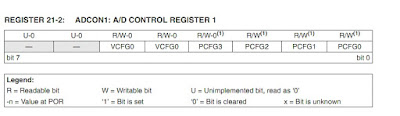ADCON1 Register

Basically ADC is like as voltage divider . According to voltage It produce output .

bit 5 : VCFG0: Voltage Reference Configuration bit (VREF- source)
1 = VREF- (AN2)
0 = VSS or 0 volt

bit 4 :VCFG0: Voltage Reference Configuration bit (VREF+ source)
1 = VREF+ (AN3)
0 = VDD or 5volt

We will set  VCFG0[bit 5]=0and VCFG0[bit4]=0 . So we will get highest value 5volt and lowest value 0volt.The ADCON1 is a 10 bit register that means  2 to the power 10  is it's highest counting capacity and result is 1024 . So this register can count from 0 to 1023 . When 0 volt , we get reading at RA0 pin  0 .When 5 volt , we get reading at RA0 pin 1023. It means 5volt equivalent to 1023 .

If  1023 reading value    equal   to 5 volt.
So 1      reading value    equal   to  5/1023 volt

## Source Code :

``````

void main() {
int source=0;
ADCON1=0x0E;                 // Configuring RA0 pin as input
CMCON=7;
TRISB=0x00;
PORTB.F0=0;
PORTB.F1=0;
PORTB.F2=0;
PORTB.F3=0;
PORTB.F4=0;

while(1){

PORTB.F0=0;
PORTB.F1=0;
PORTB.F2=0;
PORTB.F3=0;
PORTB.F4=1;
}
PORTB.F0=0;
PORTB.F1=0;
PORTB.F2=0;
PORTB.F3=1;
PORTB.F4=1;
}
PORTB.F0=0;
PORTB.F1=0;
PORTB.F2=1;
PORTB.F3=1;
PORTB.F4=1;
}
PORTB.F0=0;
PORTB.F1=1;
PORTB.F2=1;
PORTB.F3=1;
PORTB.F4=1;
}
PORTB.F0=1;
PORTB.F1=1;
PORTB.F2=1;
PORTB.F3=1;
PORTB.F4=1;
}
}
}``````

## Circuit :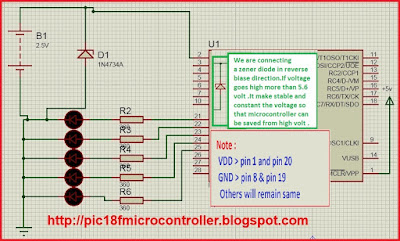circuit

## Digital Voltmeter project Using Microcontroller

Digital Voltmeter measure voltage between two point in circuit . Now we are going to make a digital voltmeter which can measure 50 volt maximum . We will use pic18f2550 microcontroller and it's ADC pin . Remember that an ADC channel of pic18f2550 can count from 0 to 1023 . Because it's ADC channel register is 10bits. As we like to  measure 50 volt , we need to apply a simple technique . So we have to convert 50 volt to 5 volt  and we get reading 1023 at adc channel  for 50 volt .
For converting we will connect 68k ohm, 22k ohm and 10k ohm resistors in serial so that during applying 50 volt it makes 5 volt drop at 10k ohm resistor . It's the main theme .

### ADC(Analog to Digital Converter ) :

We need a basic knowledge about ADC . Let's  take a look at here :ADCON1 Register

Basically ADC is like as voltage divider . According to voltage It produce output .

bit 5 : VCFG0: Voltage Reference Configuration bit (VREF- source)
1 = VREF- (AN2)
0 = VSS or 0 volt

bit 4 :VCFG0: Voltage Reference Configuration bit (VREF+ source)
1 = VREF+ (AN3)
0 = VDD or 5volt

We will set  VCFG0[bit 5]=0and VCFG0[bit4]=0 . So we will get highest value 5volt and lowest value 0volt.The ADCON1 is a 10 bit register that means  2 to the power 10  is it's highest counting capacity and result is 1024 . So this register can count from 0 to 1023 . When 0 volt , we get reading at RA0 pin  0 .When 5 volt , we get reading at RA0 pin 1023. It means 5volt equivalent to 1023 .

If  1023 reading value    equal   to 50 volt.
So 1      reading value    equal   to  50/1023 volt

### Source Code :

``````

sbit LCD_RS at LATB7_bit;
sbit LCD_EN at LATB6_bit;
sbit LCD_D4 at LATB5_bit;
sbit LCD_D5 at LATB4_bit;
sbit LCD_D6 at LATB3_bit;
sbit LCD_D7 at LATB2_bit;

sbit LCD_RS_Direction at TRISB7_bit;
sbit LCD_EN_Direction at TRISB6_bit;
sbit LCD_D4_Direction at TRISB5_bit;
sbit LCD_D5_Direction at TRISB4_bit;
sbit LCD_D6_Direction at TRISB3_bit;
sbit LCD_D7_Direction at TRISB2_bit;
char volt[]="        ";
char mv[]="v";
void main() {
double source=0;
float calv=0;
float sum=0;
float mult=0;
ADCON1=0x0E;                 // Configure RA) pin as input
CMCON=7;
Lcd_Init();                        // Initialize LCD
Lcd_Cmd(_LCD_CLEAR);               // Clear display
Lcd_Cmd(_LCD_CURSOR_OFF);
Lcd_Out(2,15,mv);
while(1){
calv=(source*50.50)/1023;
floattostr(calv,volt);
Lcd_Out(1,1,"Volt Meter");
Lcd_Out(2,1,"V=");
Lcd_Out(2,4,volt);
}
}

``````

## Thief Detector using Microcontroller & PIR Motion Sensor

For home security , we can build  " Thief Detector "  using  PIC 18f2550 microcontroller and PIR Motion Sensor . When something will be moving front to the sensor , the thief detector system will give an alarm to us . That's the basic theme of this project .

### PIR Motion Sensor :

Actually this sensor is continuously transmitting an Infrared Ray signal and receiving the reflected  signal . According to the information of  receiving signal it changes it's output signal . You can see the pin out of PIR Motion Sensor .
Generally  when something moving in the front of it  , the sensor produce 3.3 volt to it's out pin . Otherwise the out pin will be 0 volt . By applying this technique we can get information or status of out doors .
We will get the PIR Sensor connected with ADC(Analog to Digital Converter ) pin of PIC 18F2550 . We will use RA0 as input .

### ADC(Analog to Digital Converter ) :

We need a basic knowledge about ADC . Let's  take a look at here :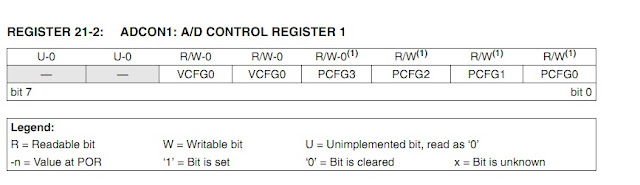ADCON1 Register

Basically ADC is like as voltage divider . According to voltage It produce output .

bit 5 : VCFG0: Voltage Reference Configuration bit (VREF- source)
1 = VREF- (AN2)
0 = VSS or 0 volt

bit 4 :VCFG0: Voltage Reference Configuration bit (VREF+ source)
1 = VREF+ (AN3)
0 = VDD or 5volt

We will set  VCFG0[bit 5]=0and VCFG0[bit4]=0 . So we will get highest value 5volt and lowest value 0volt.The ADCON1 is a 10 bit register that means  2 to the power 10  is it's highest counting capacity and result is 1024 . So this register can count from 0 to 1023 . When 0 volt , we get reading at RA0 pin  0 .When 5 volt , we get reading at RA0 pin 1023. It means 5volt equivalent to 1023 .

If  5 volt    equal    reading 1023 .
So 1  volt   equal    reading 1023/5  [When something detect PIR Sensor provide 3.3 volt at Output Pin]
So 3.3 volt equal  reading  (1023/5)*3.3 =675.8 . When we get reading 675 at ADC channel  , we understand  that sensor detects something . So it make PORTB.F6 pin high and Buzzer turns on .

### Source Code :

``````

void main() {
int  input;
CMCON=7;
TRISB.F6=0;
while (1) {
if(input>=675){
PORTB.F6=1;
delay_ms(4000);
}
PORTB.F6=0;
}
}

``````

## Thank You !!

Ain't getting any visitors!

## Featured Post

### Rs 232 , Serial Communication with PIC Microcontroller in Proteus [step by step details]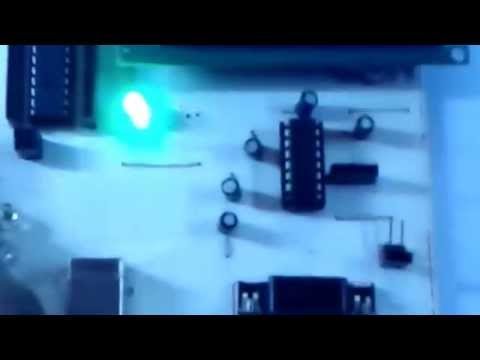## Tags

: (1) 18F2550 (1) 36KHz (3) and (1) arduino (1) Based (1) battery (1) Bipolar (1) Blinking (1) blinks (1) Bluetooth (1) button (1) circuit (1) clock (1) control (1) Db9 (1) DC Motor (2) digital (2) display (2) DS1307 (1) electronic (1) flash (1) flashing (1) HC-06 (1) home (1) how (1) How to (10) i2c tutorial (1) in (1) indicator (1) interface (8) interfacing (3) Interrupt (3) Introduction (1) IR Receiver (4) key pad (1) keyboard (1) keypad (1) lavel (1) Lcd 16x2 (2) lcd 2x16 (2) led (1) lm35 (2) LPG (1) machine (1) make (1) making (1) matrix (1) max232 (1) meter (2) microchip (4) microchips (3) mikroC (5) musical (1) NEC Protocol (4) pcb (5) PIC (3) pic controller (11) pic proteus (1) Pic Tutorial (12) pic18 (2) pic18f2550 (11) picRFモジュール (1) proteus (6) push (1) push button (1) PWM (1) real (1) Rs 232 (1) Rs232 (1) scroll (1) scrolling (1) Serial Port (1) simulation (2) step by step (7) step bystep (1) text (2) time (1) timer (4) timer0 (4) tone (1) tutorial (2) Unipolar (1) USB (1) usb 1.0 (1) USB HID (1) using (9) voltmeter (1) voting (1) with (2) work (1)

## Traffic Feed

Live Traffic Feed
Visitor Tracking